# 5 river flow measurement methods

Measure river’s flow is important for hydrological study about viability of hydropower plant installation. And to understand the behavior of water resource.

### Container’s method

It is the simplest method, uses a container with known volume $V$ and counts filling time $t$. Flow rate $Q$ in $m^3/s$ is the relation between these values.

$Q=\frac{V}{t}$

Must be done many measurements to take the average $Q_{m}$ and in various times of year.

$Q_{m}=\frac{Q_{1}+Q_{2}+...+Q_{n}}{n}$

This method can only be applied in streams.

### Measurement with floater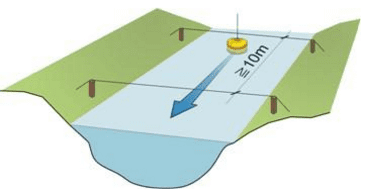Consists in put a floater to go through a part of river. This method is also used to measure surface’s speed, it’s higher than average speed. Steps to calculate flow rate are:

1. Choose a river stretch, must be the most straight as possible and constant transversal section.
2. Measure river stretch $L$.
3. Measure transversal section area $A$. To obtain this value, it is necessary to use bathymetry. Which is depth measurement of rivers, seas and lakes. It is represented by bathymetric curves, which show areas of same depth like curve levels.
4. Put a floater to go through the chosen stretch and to count the time $t$.
5. Calculate surface speed $V$.

$V=\frac{L}{t}$

Usually, average speed is 80% of surface speed. Therefore, flow rate formula in $m^3/s$ is:

$Q=0,8\cdot V\cdot A$

### Measurement with current meter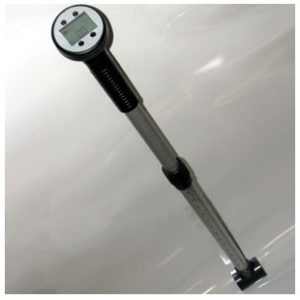This is the most convenient method, uses an instrument with a propeller in an extremity. Propeller stays underwater and pointed to opposite direction of river current. Propeller’s rotation is directly proportional to flow speed, which is indicated in other extremity. Of course that must make measurements in many points and different depths to obtain average. Flow rate $Q$ formula is:

$Q=\sum A_{i}\cdot V_{i}$

$A_{i}$ is area of each section, where speed is $V_{i}$. While $\sum$ is the sum of multiplication area times velocity. This method has high precision.

Is the abbreviation of Acoustic Doppler Current Profiler. This equipment uses Doppler effect to measure river’s speed. Emits ultrasonic pulses in fixed frequency, suspended particles move at same speed as river’s speed. When a particle approaches ADCP, the received frequency by instrument is higher and it goes away, frequency decreases in relation to ADCP.

Below, the Doppler effect formula to calculate speed of moving object, considering stationary detector and moving object.

$f_{D}=f\frac{v}{v\pm v_{S}}$

• $f_{D}$ is frequency detected by sensor.
• $f$ is frequency of transmitted sound wave.
• $v$ is sound speed in water.
• $v_{S}$ is speed of suspended particle.

The ADCP calculates flow rate with a software and can be installed in floaters, boats and underwater structures.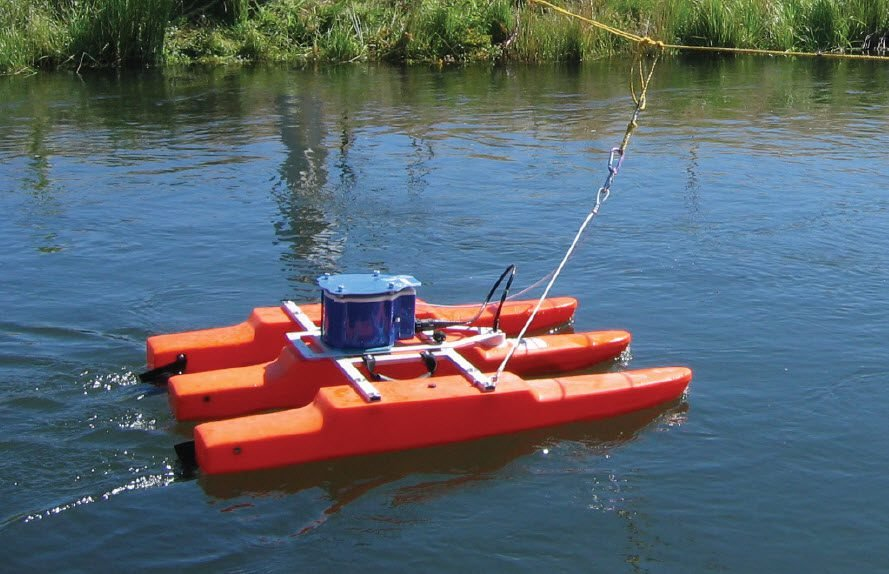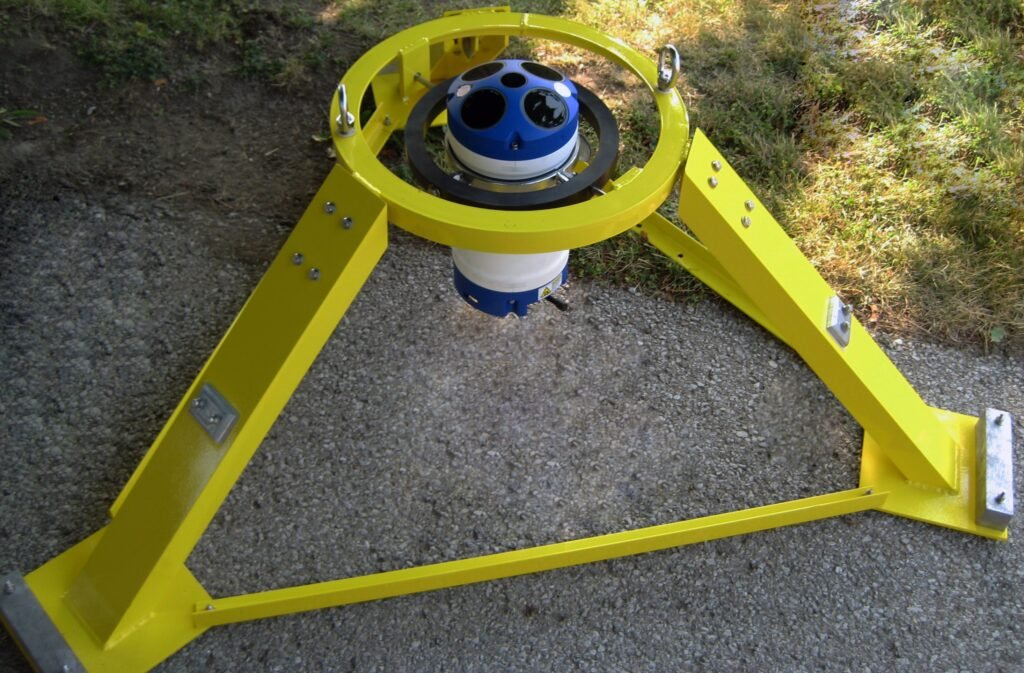It’s highly precise and saves time, however it is expensive and requires specialized labor for operation.

### Measurement with spillway

The spillway is a small dam with a window to flow water. Flow rate calculation depends on opening shape. In this post is shown the most common spillways.

#### Rectangular spillway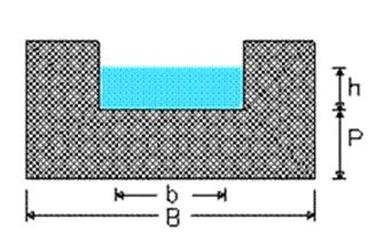Flow rate in a rectangular spillway is:

$Q=1,838(b-0,2h)h^{3/2}$

#### Triangular spillway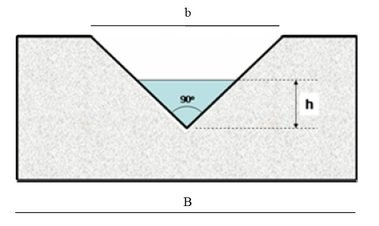$Q=1,4h^{5/2}$

#### Trapezoidal spillway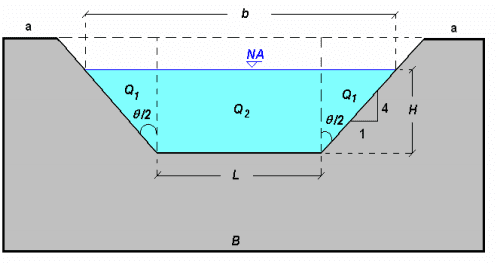$Q=1,9\cdot b\cdot H^{3/2}$